# How to sum negative and positive values using GroupBy in Pandas?

In this article, we will discuss how to calculate the sum of all negative numbers and positive numbers in DataFrame using the GroupBy method in Pandas.

To use the groupby() method use the given below syntax.

Syntax: df.groupby(column_name)

### Stepwise Implementation

Step 1: Creating lambda functions to calculate positive-sum and negative-sum values.

```pos = lambda col : col[col > 0].sum()
neg = lambda col : col[col < 0].sum()```

Step 2: We will use the groupby() method and apply the lambda function to calculate the sum.

```d = df.groupby(df['Alphabet'])
print(d['Frequency'].agg([('negative_values', neg),
('positive_values', pos)
]))
print(d['Bandwidth'].agg([('negative_values', neg),
('positive_values', pos)
]))```

### Examples

Example 1:

Calculate the sum of all positive as well as negative values of a, b, c for both columns i.e., Frequency and bandwidth

## Python3

 `# Import Necessary Libraries ` `import` `pandas as pd ` `import` `numpy as np ` ` `  `# Creating a DataFrame with  ` `# random values ` `df ``=` `pd.DataFrame({``'Alphabet'``: [``'a'``, ``'b'``, ``'c'``, ``'c'``, ` `                                ``'a'``, ``'a'``, ``'c'``, ``'b'``], ` `                    `  `                   ``'Frequency'``: [``-``10``, ``29``, ``-``12``, ``-``190``, ` `                                 ``72``, ``-``98``, ``-``12``, ``0``], ` `                    `  `                   ``'BandWidth'``: [``10``, ``34``, ``23``, ``-``10``, ``-``87``, ` `                                 ``-``76``, ``365``, ``10``]}) ` ` `  `print``(df) ` ` `  `# Group By dataframe on categorical ` `# values ` `d ``=` `df.groupby(df[``'Alphabet'``]) ` ` `  `# creating lambda function to calculate ` `# positive as well as negative values ` `def` `pos(col):  ` `  ``return` `col[col > ``0``].``sum``() ` ` `  `def` `neg(col):  ` `  ``return` `col[col < ``0``].``sum``() ` ` `  ` `  `# Apply lambda function to particular  ` `# column ` `print``(d[``'Frequency'``].agg([(``'negative_values'``, neg), ` `                          ``(``'positive_values'``, pos) ` `                          ``])) ` ` `  `print``(d[``'Bandwidth'``].agg([(``'negative_values'``, neg), ` `                          ``(``'positive_values'``, pos) ` `                          ``])) `

Output: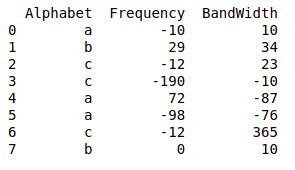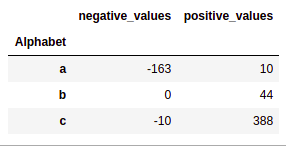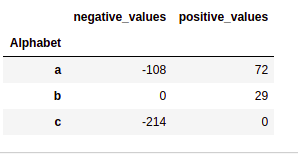Example 2:

Calculate the sum of all positive as well as negative values of a, b for both columns i.e., X and Y

## Python3

 `# Import Necessary Libraries ` `import` `pandas as pd ` `import` `numpy as np ` ` `  `# Creating a DataFrame with random values ` `df ``=` `pd.DataFrame({``'Function'``: [``'F(x)'``, ``'F(x)'``, ``'F(y)'``, ` `                                ``'F(x)'``, ``'F(y)'``, ``'F(x)'``, ` `                                ``'F(x)'``, ``'F(y)'``], ` `                    `  `                   ``'X'``: [``-``10``, ``29``, ``-``12``, ``-``190``, ``72``, ``-``98``, ` `                         ``-``12``, ``0``], ` `                    `  `                   ``'Y'``: [``10``, ``34``, ``23``, ``-``10``, ``-``87``, ``-``76``,  ` `                         ``365``, ``10``]}) ` ` `  `print``(df) ` ` `  `# Group By dataframe on categorical values ` `d ``=` `df.groupby(df[``'Function'``]) ` ` `  `# creating lambda function to calculate ` `# positive as well as negative values ` `def` `pos(col):  ` `  ``return` `col[col > ``0``].``sum``() ` ` `  `def` `neg(col):  ` `  ``return` `col[col < ``0``].``sum``() ` ` `  `# Apply lambda function to particular  ` `# column ` `print``(d[``'X'``].agg([(``'negative_values'``, neg), ` `                  ``(``'positive_values'``, pos) ` `                  ``])) ` ` `  `print``(d[``'Y'``].agg([(``'negative_values'``, neg), ` `                  ``(``'positive_values'``, pos) ` `                  ``])) `

Output: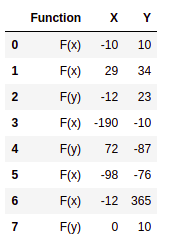DataFrame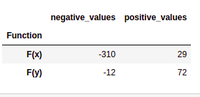X Output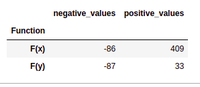Y Output

Example 3:

Calculate the sum of all positive as well as negative values of every name i.e., Marks. The next step is to make the lambda function to calculate the sum. In the last step, we will group the data according to the names and call the lambda functions to calculate the sum of the values.

## Python3

 `# Import Necessary Libraries ` `import` `pandas as pd ` `import` `numpy as np ` ` `  `# Creating a DataFrame with random values ` `df ``=` `pd.DataFrame({``'Name'``: [``'Aryan'``, ``'Nityaa'``, ``'Dhruv'``, ` `                            ``'Dhruv'``, ``'Nityaa'``, ``'Aryan'``, ` `                            ``'Nityaa'``, ``'Aryan'``, ``'Aryan'``,  ` `                            ``'Dhruv'``, ``'Nityaa'``, ``'Dhruv'``,  ` `                            ``'Dhruv'``], ` `                   ``'Marks'``: [``90``, ``93``, ``78``, ``56``, ``34``, ``12``, ``67``,  ` `                             ``45``, ``78``, ``92``, ``29``, ``88``, ``81``]}) ` `print``(df) ` ` `  `# Group By dataframe on categorical values ` `d ``=` `df.groupby(df[``'Name'``]) ` ` `  `# creating lambda function to calculate ` `# positive as well as negative values ` `def` `pos(col):  ` `  ``return` `col[col > ``0``].``sum``() ` ` `  `def` `neg(col):  ` `  ``return` `col[col < ``0``].``sum``() ` ` `  ` `  `# Apply lambda function to particular ` `# column ` `print``(d[``'Marks'``].agg([(``'negative_values'``, neg), ` `                      ``(``'positive_values'``, pos) ` `                      ``])) `

Output: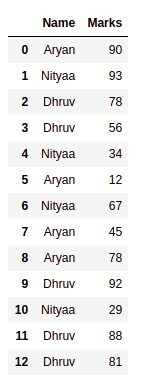Names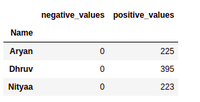Marks

Whether you're preparing for your first job interview or aiming to upskill in this ever-evolving tech landscape, GeeksforGeeks Courses are your key to success. We provide top-quality content at affordable prices, all geared towards accelerating your growth in a time-bound manner. Join the millions we've already empowered, and we're here to do the same for you. Don't miss out - check it out now!

Previous
Next